Semiconductor Diode MCQ Level – 2

# Semiconductor Diode MCQ Level – 2 - IIT JAM

Test Description

## 10 Questions MCQ Test - Semiconductor Diode MCQ Level – 2

Semiconductor Diode MCQ Level – 2 for IIT JAM 2023 is part of IIT JAM preparation. The Semiconductor Diode MCQ Level – 2 questions and answers have been prepared according to the IIT JAM exam syllabus.The Semiconductor Diode MCQ Level – 2 MCQs are made for IIT JAM 2023 Exam. Find important definitions, questions, notes, meanings, examples, exercises, MCQs and online tests for Semiconductor Diode MCQ Level – 2 below.
Solutions of Semiconductor Diode MCQ Level – 2 questions in English are available as part of our course for IIT JAM & Semiconductor Diode MCQ Level – 2 solutions in Hindi for IIT JAM course. Download more important topics, notes, lectures and mock test series for IIT JAM Exam by signing up for free. Attempt Semiconductor Diode MCQ Level – 2 | 10 questions in 45 minutes | Mock test for IIT JAM preparation | Free important questions MCQ to study for IIT JAM Exam | Download free PDF with solutions
 1 Crore+ students have signed up on EduRev. Have you?
Semiconductor Diode MCQ Level – 2 - Question 1

### The voltage across a diode in a full-wave rectifier having input voltage of peak value Vm during its non-conducting period is :

Detailed Solution for Semiconductor Diode MCQ Level – 2 - Question 1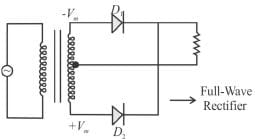During non-conducting period, the voltage across D1 is Vm. = -2Vm.
The correct answer is: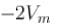Semiconductor Diode MCQ Level – 2 - Question 2

### When reverse voltage across silicon diode is changed from 4V to 8 V, what happens to depletion layer?

Detailed Solution for Semiconductor Diode MCQ Level – 2 - Question 2

Under reverse bias (applying a negative voltage to the P-side with respect to the N-side), the potential drop (i.e., voltage) across the depletion region increases. Essentially, majority carriers are pushed away from the junction, leaving behind more charged ions.

Semiconductor Diode MCQ Level – 2 - Question 3

### When two identical zener diodes are connected in series, what happens to the breakdown voltage of the combination ?

Detailed Solution for Semiconductor Diode MCQ Level – 2 - Question 3

The two zener diodes when connected in series will double the breakdown voltage.

Semiconductor Diode MCQ Level – 2 - Question 4

For the clamping network shown below, the resulting output for the applied input will be.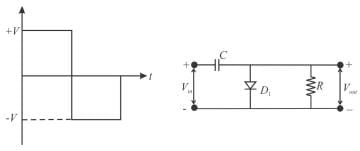Detailed Solution for Semiconductor Diode MCQ Level – 2 - Question 4

For the first position half of the input signal, the current pass through the diode, hence no current flows through the resistor R, hence, no output voltage is observed and capacitor is charged upto voltage V. For second half (negative), diode blocks the flow of current, hence current pass through resistor R but in opposite direction, hence the potential difference observed across R comes input from signal-V and from the capacitor again in opposite direction, thus making it-2V.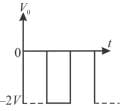Semiconductor Diode MCQ Level – 2 - Question 5

An avalanche effect is observed in a diode when

Detailed Solution for Semiconductor Diode MCQ Level – 2 - Question 5

The avalanche effect is observed when reverse biased voltage is more than the breakdown voltage.
The correct answer is: the reverse voltage exceeds the breakdown voltage

Semiconductor Diode MCQ Level – 2 - Question 6

A sinusoidal input voltage Vin of frequency ω is fed to the circuit shown in the figure where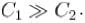if Vm the Peak value of the input voltage, then output voltage (Vout) is.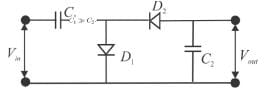Detailed Solution for Semiconductor Diode MCQ Level – 2 - Question 6

The circuit looks like a voltage doubler. At the peak of positive half cycle D1 is forward biased and D2 is reverse biased. Ideally, this charges C1 to the peak voltage Vm , with polarity as shown below. At the peak of the negative half cycle, D1 is reverse biased and D2 is forward biased. Because the source and C1 are in series, C2 will try to change towards 2Vm
The correct answer is: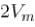Semiconductor Diode MCQ Level – 2 - Question 7

An ac voltage of 220 Vrms is applied to the primary of a 10:1 step-down transformer. The secondary of the transformer is centre tapped and connected to a full-wave rectifier with a load resistance. This dc voltage appearing in the load is.

Detailed Solution for Semiconductor Diode MCQ Level – 2 - Question 7

For step-down transformer,
E2 < E1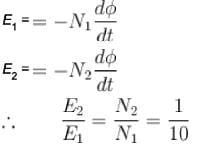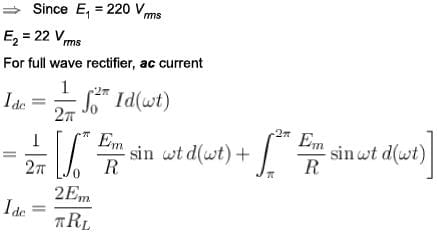(44/π)

Semiconductor Diode MCQ Level – 2 - Question 8

The value of I and V for the circuit shown below are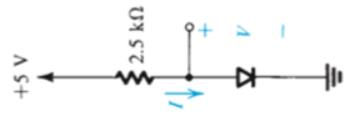Detailed Solution for Semiconductor Diode MCQ Level – 2 - Question 8

I = V / R or 5 / 2.5 or 2A
For an ideal diode when it is conducting the potential drop across its terminal is zero

Semiconductor Diode MCQ Level – 2 - Question 9

A p-n diode is reverse biased. The resistance measured by an ohm-meter connected across it will be.

Detailed Solution for Semiconductor Diode MCQ Level – 2 - Question 9

In case of reverse biased, the resistance of an ideal p-n diode should be infinity. But practically infinite is not possible and so resistance becomes very high.

Semiconductor Diode MCQ Level – 2 - Question 10

Which graph represents the nature of the curve between charge density p and distance r near the depletion region of p-n junction diode?

Detailed Solution for Semiconductor Diode MCQ Level – 2 - Question 10

Holes will diffuse to right and electrons to left junction initially. Positive holes neutralize the acceptor ions near the function in the p-type silicon and disappeared across junction. Similarly, electrons will be neutralized across n-type by combination with holes of p-type due to diffusion. Thus, across p-type and n-type, there will be lack of majority charge carriers and excess of minority charge carriers. Then, majority charge carriers decreases as we move away from junction.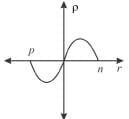(Scan QR code)